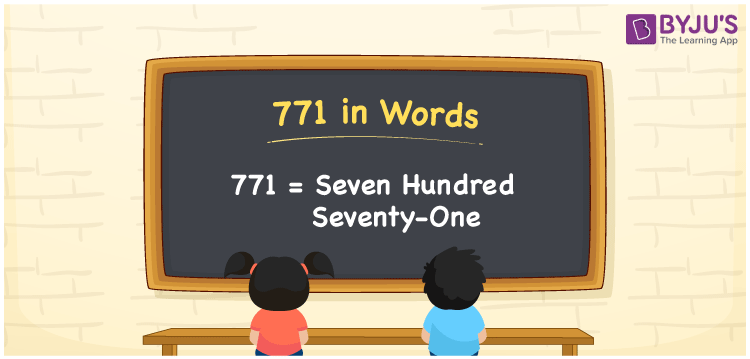# 771 in Words

Seven hundred seventy-one is used to express the number 771. In Mathematics, 771 is a natural number and it has three digits. Also, 771 is said to be a cardinal number, as it is used to express the specific quantity. For example, the cost of 10 pens is Rs. 771. In this article, we are going to learn the procedure of writing the number 771 in words using the place value system in detail.

 771 in Words: Seven Hundred Seventy-one. Seven Hundred Seventy-one in Numerical Form: 771.

## 771 in English Words## How to Write 771 in Words?

The place values of 771 are presented in the below table.

 Hundreds Tens Ones 7 7 1

The expanded form of 771 is as follows:

= 7 × Hundred + 7 × Ten + 1 × One

= 7 × 100 + 7 × 10 + 1 × 1

= 700 + 70 + 1

= 771

= Seven hundred seventy-one

Hence, 771 in words is seven hundred seventy-one.

After 770 but before 772, the natural number 771 exists.

771 in words – Seven hundred seventy-one

Is 771 an odd number? – Yes

Is 771 an even number? – No

Is 771 a perfect square number? – No

Is 771 a perfect cube number? – No

Is 771 a prime number? – No

Is 771 a composite number? – Yes

## Frequently Asked Questions on 771 in Words

Q1

### Write 771 in words.

771 in words is seven hundred seventy-one.

Q2

### Simplify 700 + 71, and express it in words.

Simplifying 700 + 71, we get 771. Hence, 771 in words is seven hundred seventy-one.

Q3

### Is 771 an odd number?

Yes, 771 is an odd number.